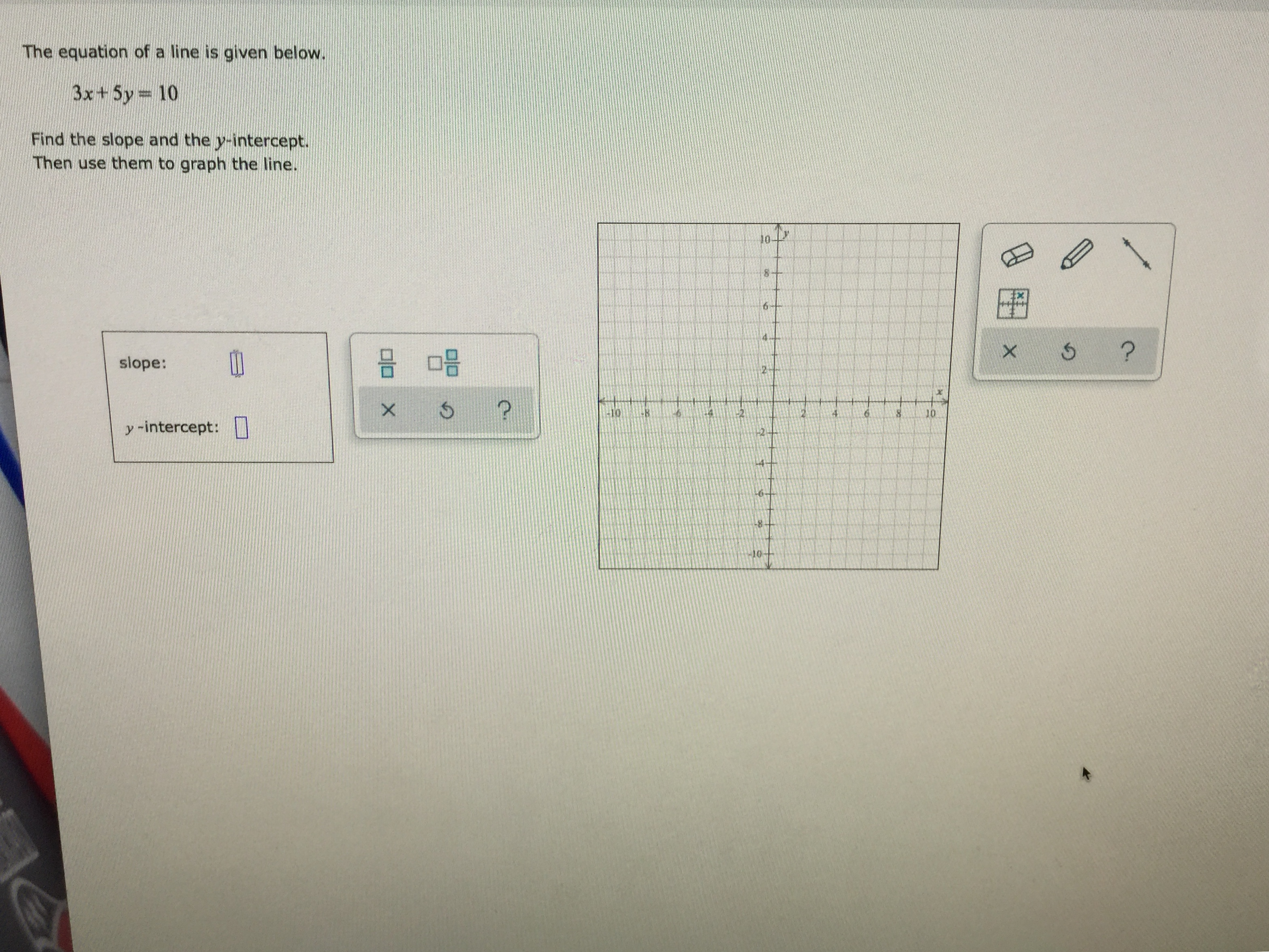# The equation of a line is given below.3x+ 5y 10Find the slope and the y-intercept.Then use them to graph the line.86Xslope:?X10104y -intercept:10-B

Question
12 viewshelp_outlineImage TranscriptioncloseThe equation of a line is given below. 3x+ 5y 10 Find the slope and the y-intercept. Then use them to graph the line. 8 6 X slope: ? X 10 10 4 y -intercept: 10- B fullscreen
check_circle

Step 1

Given equation of line is :

We need to find the slope and y-intercept of the line.

Also graph this line.

Step 2

Firstly we write the given equation into the form of slope intercept form of line.

Standard slope intercept form of line is :

Here m is slope of the line

b is y-intercept of the line.

Step 3

Now write the line in this form:

Solve...

### Want to see the full answer?

See Solution

#### Want to see this answer and more?

Solutions are written by subject experts who are available 24/7. Questions are typically answered within 1 hour.*

See Solution
*Response times may vary by subject and question.
Tagged in

### Equations and In-equations